### IF Function in MS Excel

The IF Function in MS Excel can be used in many ways such as:
• IF Function only
• IF Function with AND/OR Criteria
• Nested IF (Using Multiple IF at same time)

How to use IF function
The IF function checks whether a condition is met, and returns one value if the condition is TRUE, or another value if the condition is FALSE.
Let's learn with Example
For example, if D2 is less than or equal to 40 then admissions are open , otherwise Admissions are not openso E2 equals =IF(D2<=40, "YES", "NO")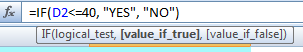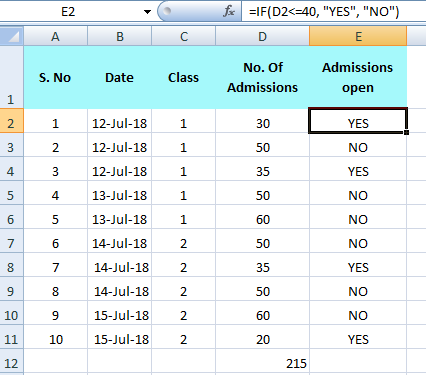Here, we have chosen the operator (less than or equal to <=), you can use other comparison operators like
• (greater than or equal to >=)
• (equal to = )
• (greater than >)
• (less than < )
• (not equal to <> ).
How to use IF function with AND/OR Criteria
• Use IF function with AND Criteria
When using the AND function, all conditions within the AND function must be TRUE for the condition to be met. The AND function can be combined with IF function to allow testing for multiple conditions.
Let's learn with Example
For example, The AND function returns TRUE if the first value B2 (points earned) is greater than or equal to 400 and the second value C2 (Sales) is greater than or equal to 500, else it returns FALSE. If TRUE, the IF function returns True, if FALSE, the IF function returns False.
So here D2 equals =IF(AND(B2>=400,C2>=500),"TRUE", "FALSE")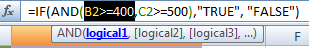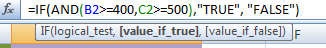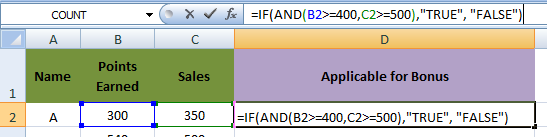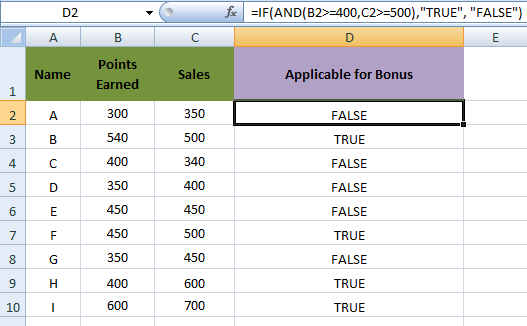• Use IF function with OR Criteria
The OR function returns TRUE if any of the conditions are TRUE and returns FALSE if all conditions are false. The OR function can be combined with IF function to allow testing for multiple conditions.
Let's learn with Example

The OR function returns TRUE if at least one value B2 (points earned) or C2 (Sales) are greater than or equal to 400 or 500, else it returns FALSE. If TRUE, the IF function returns TRUE, if FALSE, the IF function returns FALSE.
So here , D2 equals =IF(OR(B2>=400,C2>=500),"TRUE", "FALSE")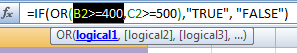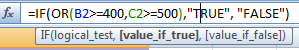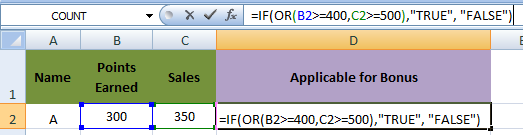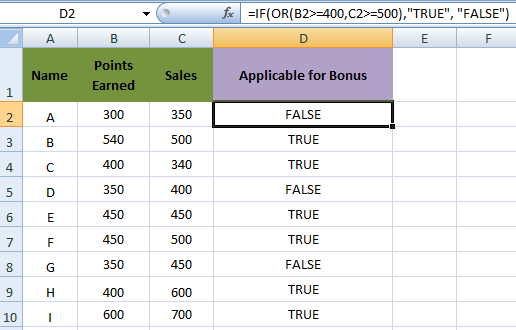• Use IF function with both AND, OR Criteria
Let's learn with Example
For example, here we will define the criteria using functions IF, AND, OR, that how a person is applicable for promotion (E2).
The AND function has two arguments B2 (points earned) or C2 (Sales) are greater than or equal to 400 or 500, OR Function has argument (Experience D2) should be greater than 5 years.
The IF function returns TRUE if both AND & OR criteria are True. The IF function returns FALSE if both AND & OR criteria are False.
So, A person is Applicable for promotion if points earned and sales are greater than 400 and 500 respectively. And Experience is greater than 5 Years.
So here E2 Equals =IF(AND(B2>400,C2>500,OR(D2>5)),"TRUE","FALSE")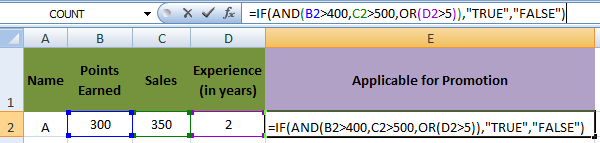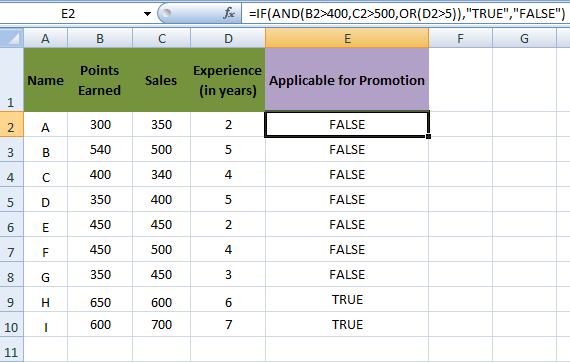You can see only Person H and I are applicable for promotion because they met all criteria.

### How to use Nested IF function (Using Multiple IF at same time)

The IF function can be nested, when you have multiple conditions to meet. The FALSE value is being replaced by another IF function to make a further test.
Let's learn with Example
For example,
If the Quantity Available(C3) is greater than or equal to 600, the nested IF formula returns Not Required.
If the Quantity Available (C3) is greater than or equal to 400, the nested IF formula returns Next Week.
If the Quantity Available (C3) is less than or equal to 200, the nested IF formula returns Order Immediately.
Else it returns False.
So Here, D3 equals
=IF(C3>=600, "Not Required", IF(C3>=400, "Next Week", IF(C3<=200, "Order Immediately")))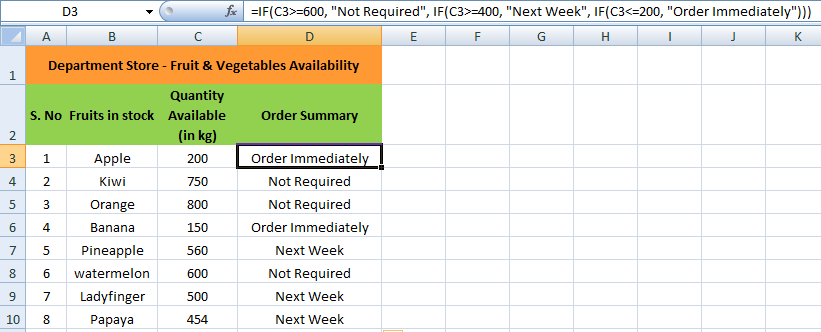Read More on Functions :

Also Read
Must read for you :